Скачать презентацию BM 410 Investments Equity Valuation 2 Calculating Intrinsic

c434de4a34d6d81b935fe8d73df46b36.ppt

• Количество слайдов: 35BM 410: Investments Equity Valuation 2: Calculating Intrinsic Value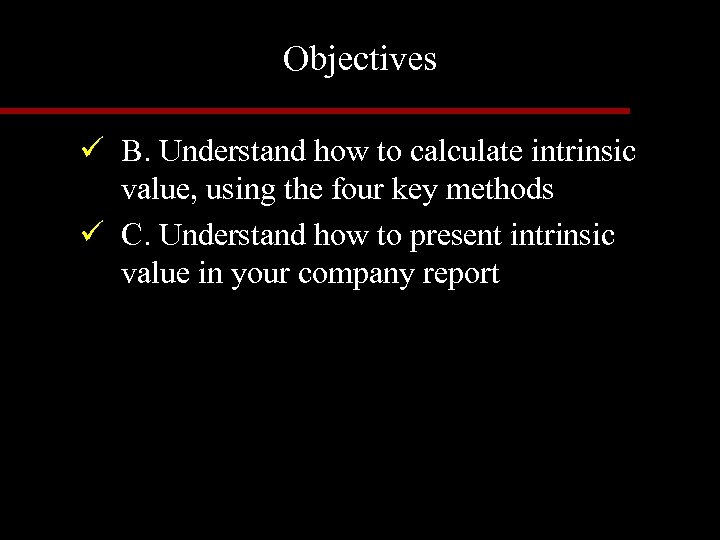Objectives ü B. Understand how to calculate intrinsic value, using the four key methods ü C. Understand how to present intrinsic value in your company report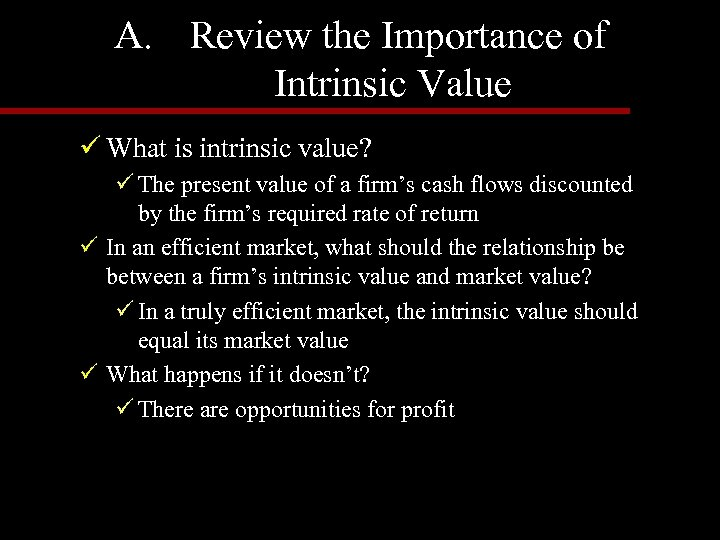A. Review the Importance of Intrinsic Value ü What is intrinsic value? ü The present value of a firm’s cash flows discounted by the firm’s required rate of return ü In an efficient market, what should the relationship be between a firm’s intrinsic value and market value? ü In a truly efficient market, the intrinsic value should equal its market value ü What happens if it doesn’t? ü There are opportunities for profit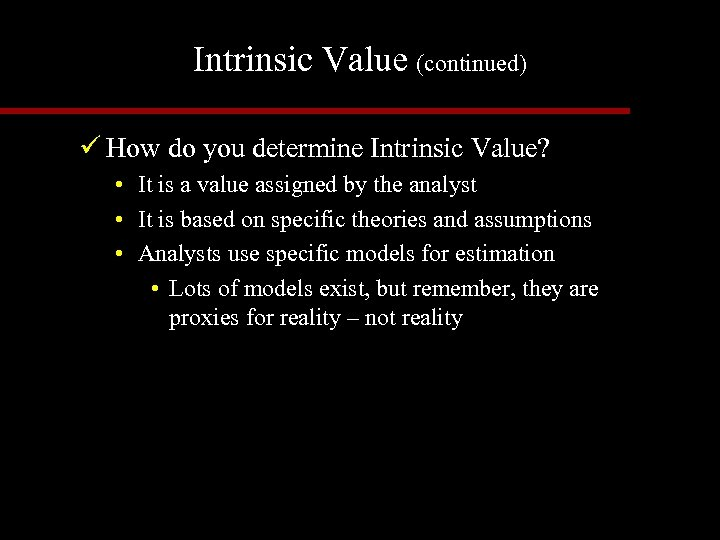Intrinsic Value (continued) ü How do you determine Intrinsic Value? • It is a value assigned by the analyst • It is based on specific theories and assumptions • Analysts use specific models for estimation • Lots of models exist, but remember, they are proxies for reality – not reality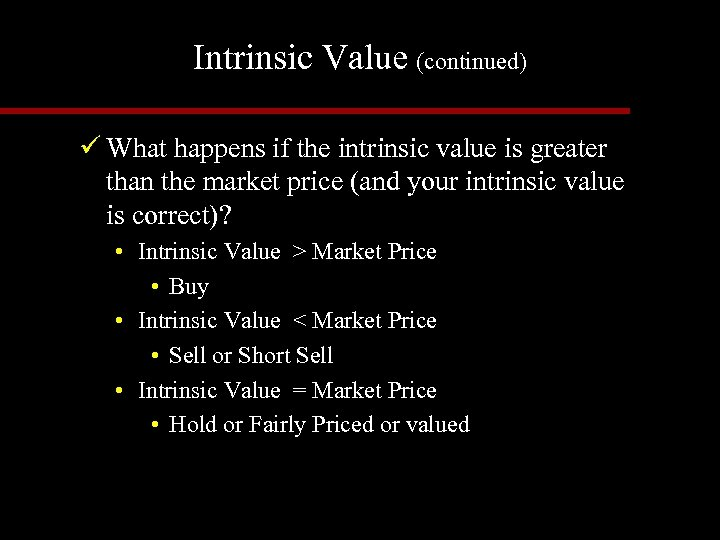Intrinsic Value (continued) ü What happens if the intrinsic value is greater than the market price (and your intrinsic value is correct)? • Intrinsic Value > Market Price • Buy • Intrinsic Value < Market Price • Sell or Short Sell • Intrinsic Value = Market Price • Hold or Fairly Priced or valued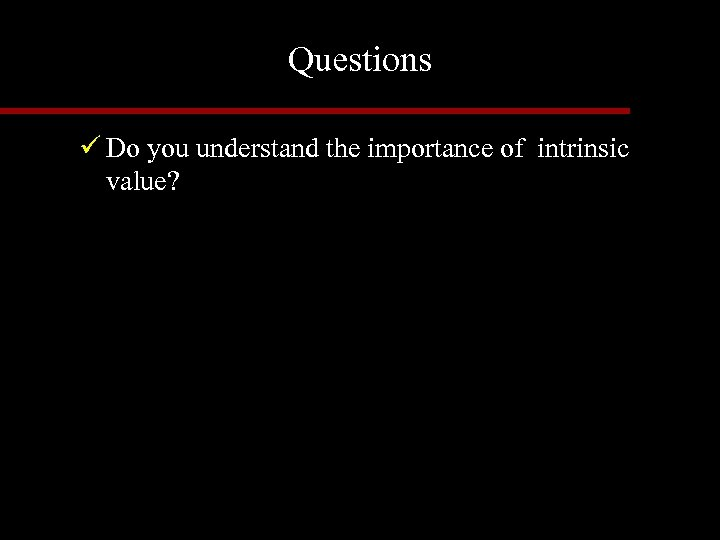Questions ü Do you understand the importance of intrinsic value?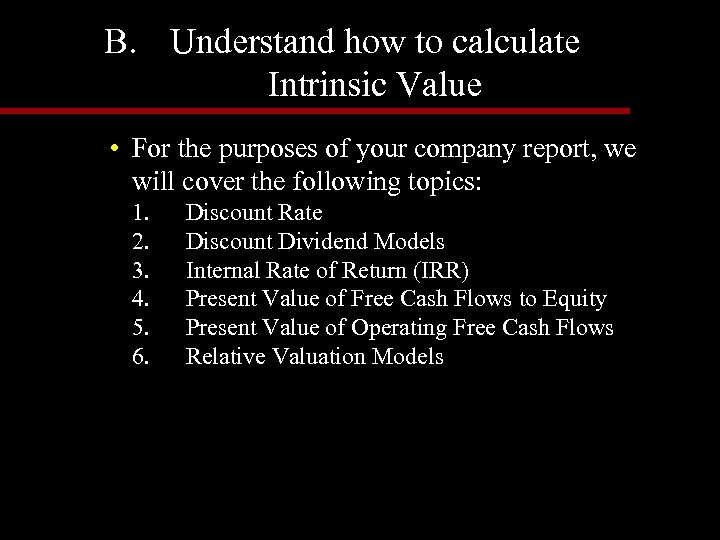B. Understand how to calculate Intrinsic Value • For the purposes of your company report, we will cover the following topics: 1. Discount Rate 2. Discount Dividend Models 3. Internal Rate of Return (IRR) 4. Present Value of Free Cash Flows to Equity 5. Present Value of Operating Free Cash Flows 6. Relative Valuation Models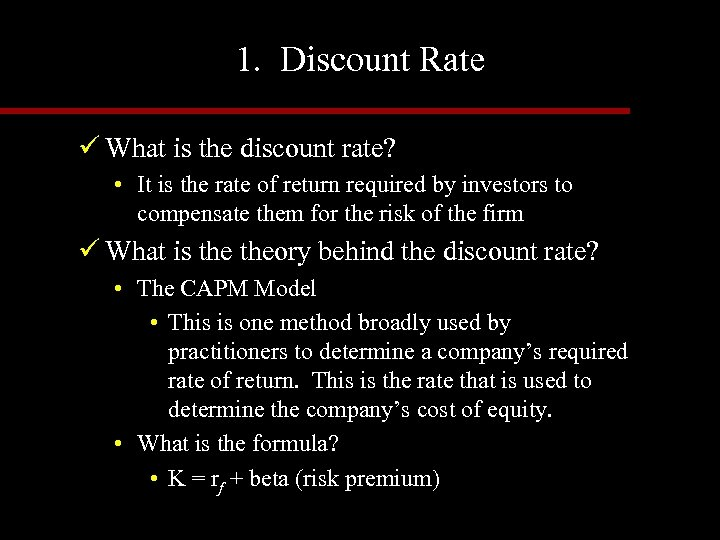1. Discount Rate ü What is the discount rate? • It is the rate of return required by investors to compensate them for the risk of the firm ü What is theory behind the discount rate? • The CAPM Model • This is one method broadly used by practitioners to determine a company’s required rate of return. This is the rate that is used to determine the company’s cost of equity. • What is the formula? • K = rf + beta (risk premium)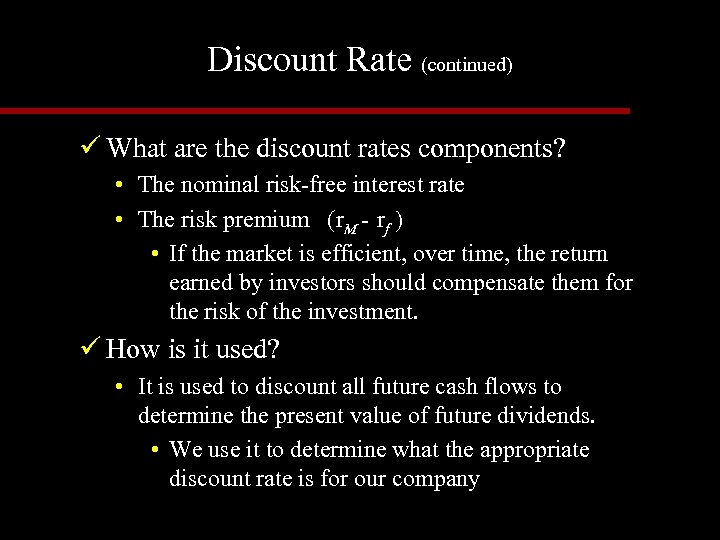Discount Rate (continued) ü What are the discount rates components? • The nominal risk-free interest rate • The risk premium (r. M - rf ) • If the market is efficient, over time, the return earned by investors should compensate them for the risk of the investment. ü How is it used? • It is used to discount all future cash flows to determine the present value of future dividends. • We use it to determine what the appropriate discount rate is for our company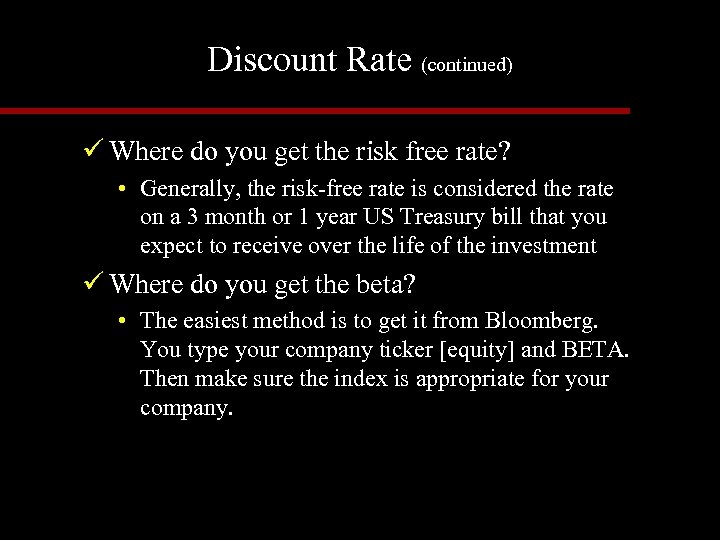Discount Rate (continued) ü Where do you get the risk free rate? • Generally, the risk-free rate is considered the rate on a 3 month or 1 year US Treasury bill that you expect to receive over the life of the investment ü Where do you get the beta? • The easiest method is to get it from Bloomberg. You type your company ticker [equity] and BETA. Then make sure the index is appropriate for your company.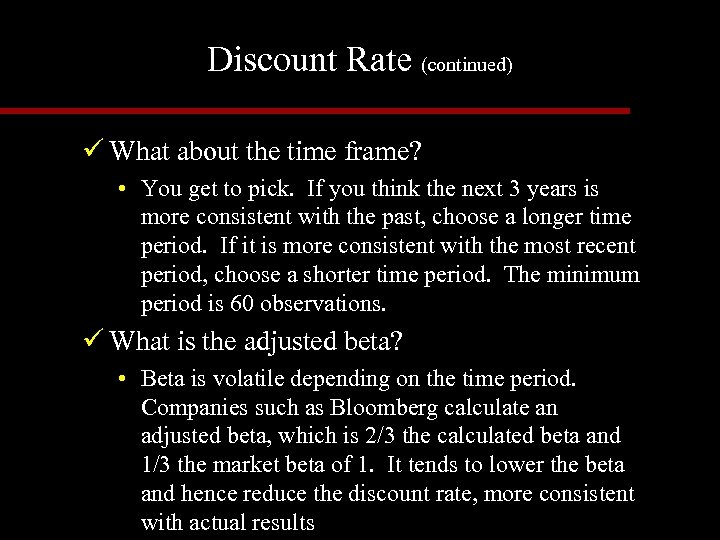Discount Rate (continued) ü What about the time frame? • You get to pick. If you think the next 3 years is more consistent with the past, choose a longer time period. If it is more consistent with the most recent period, choose a shorter time period. The minimum period is 60 observations. ü What is the adjusted beta? • Beta is volatile depending on the time period. Companies such as Bloomberg calculate an adjusted beta, which is 2/3 the calculated beta and 1/3 the market beta of 1. It tends to lower the beta and hence reduce the discount rate, more consistent with actual results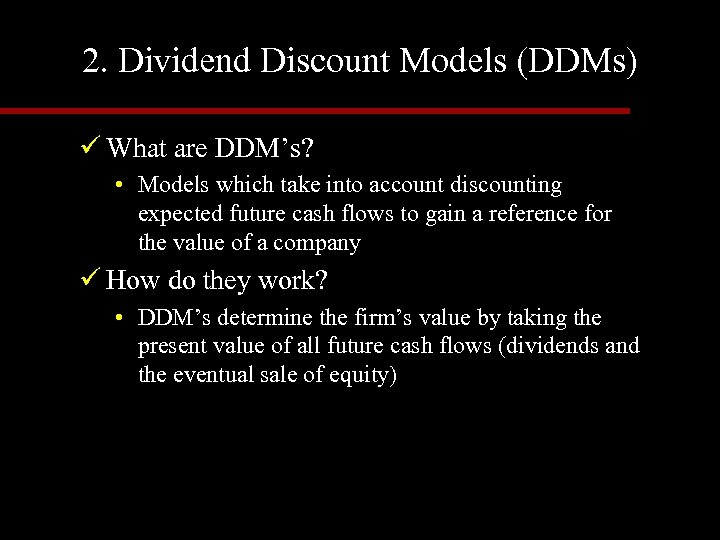2. Dividend Discount Models (DDMs) ü What are DDM’s? • Models which take into account discounting expected future cash flows to gain a reference for the value of a company ü How do they work? • DDM’s determine the firm’s value by taking the present value of all future cash flows (dividends and the eventual sale of equity)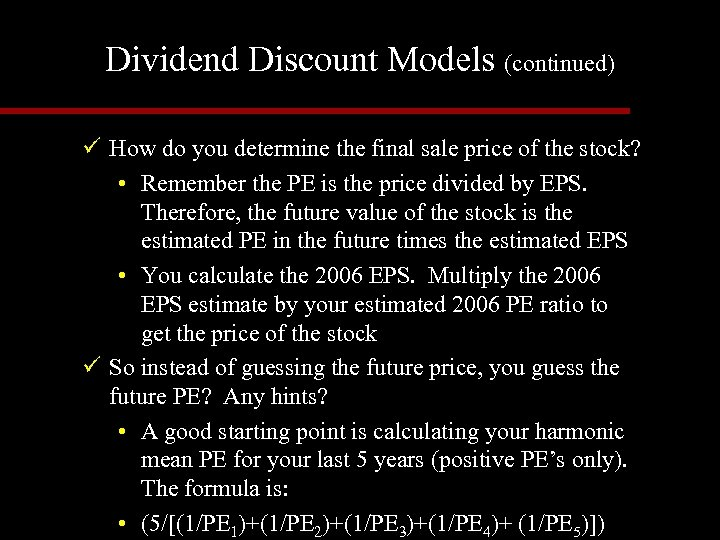Dividend Discount Models (continued) ü How do you determine the final sale price of the stock? • Remember the PE is the price divided by EPS. Therefore, the future value of the stock is the estimated PE in the future times the estimated EPS • You calculate the 2006 EPS. Multiply the 2006 EPS estimate by your estimated 2006 PE ratio to get the price of the stock ü So instead of guessing the future price, you guess the future PE? Any hints? • A good starting point is calculating your harmonic mean PE for your last 5 years (positive PE’s only). The formula is: • (5/[(1/PE 1)+(1/PE 2)+(1/PE 3)+(1/PE 4)+ (1/PE 5)])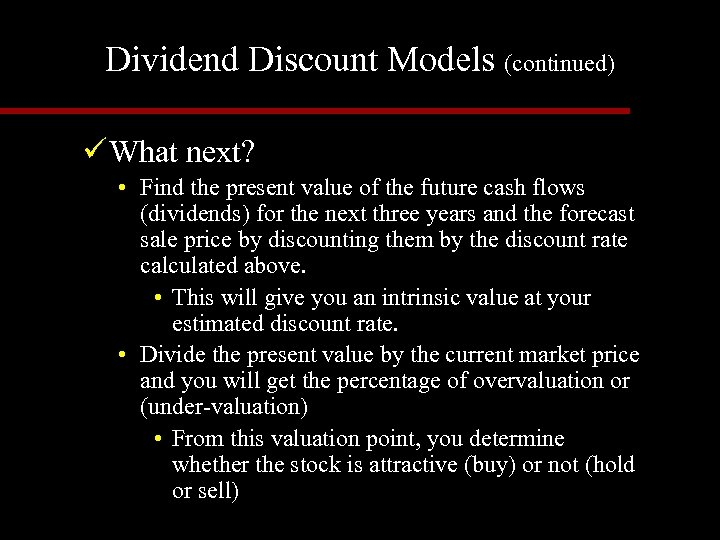Dividend Discount Models (continued) ü What next? • Find the present value of the future cash flows (dividends) for the next three years and the forecast sale price by discounting them by the discount rate calculated above. • This will give you an intrinsic value at your estimated discount rate. • Divide the present value by the current market price and you will get the percentage of overvaluation or (under-valuation) • From this valuation point, you determine whether the stock is attractive (buy) or not (hold or sell)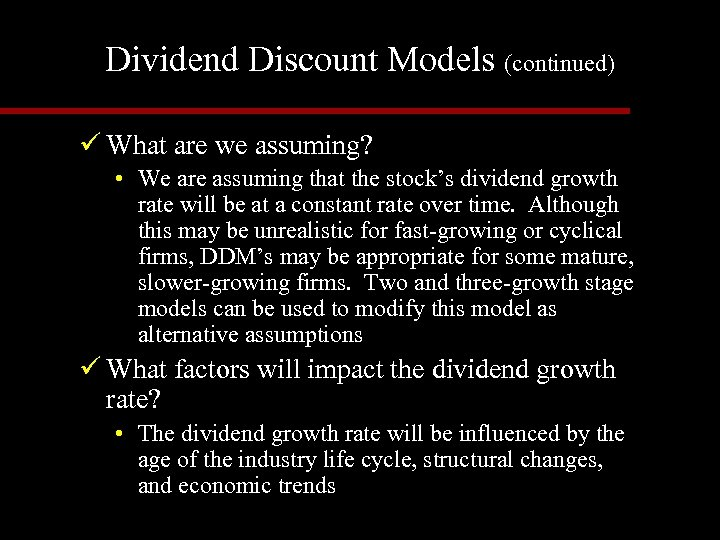Dividend Discount Models (continued) ü What are we assuming? • We are assuming that the stock’s dividend growth rate will be at a constant rate over time. Although this may be unrealistic for fast-growing or cyclical firms, DDM’s may be appropriate for some mature, slower-growing firms. Two and three-growth stage models can be used to modify this model as alternative assumptions ü What factors will impact the dividend growth rate? • The dividend growth rate will be influenced by the age of the industry life cycle, structural changes, and economic trends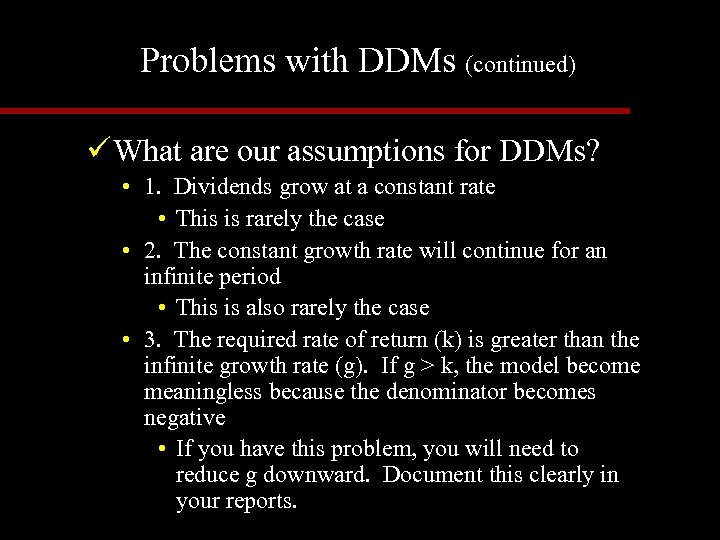Problems with DDMs (continued) ü What are our assumptions for DDMs? • 1. Dividends grow at a constant rate • This is rarely the case • 2. The constant growth rate will continue for an infinite period • This is also rarely the case • 3. The required rate of return (k) is greater than the infinite growth rate (g). If g > k, the model become meaningless because the denominator becomes negative • If you have this problem, you will need to reduce g downward. Document this clearly in your reports.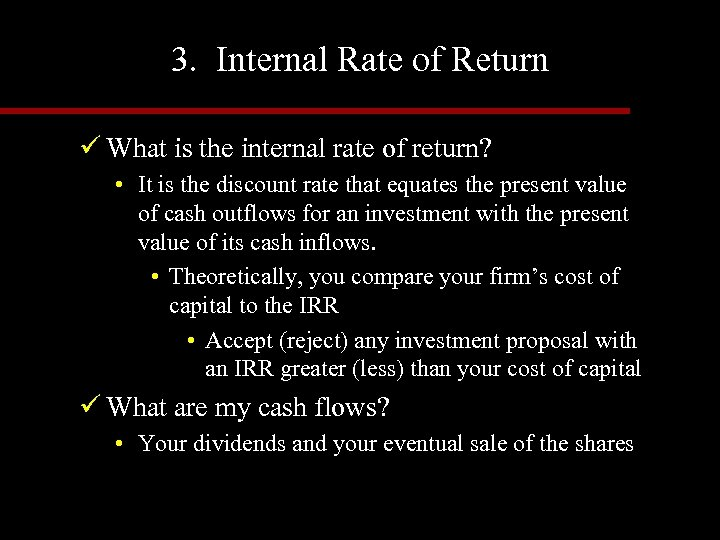3. Internal Rate of Return ü What is the internal rate of return? • It is the discount rate that equates the present value of cash outflows for an investment with the present value of its cash inflows. • Theoretically, you compare your firm’s cost of capital to the IRR • Accept (reject) any investment proposal with an IRR greater (less) than your cost of capital ü What are my cash flows? • Your dividends and your eventual sale of the shares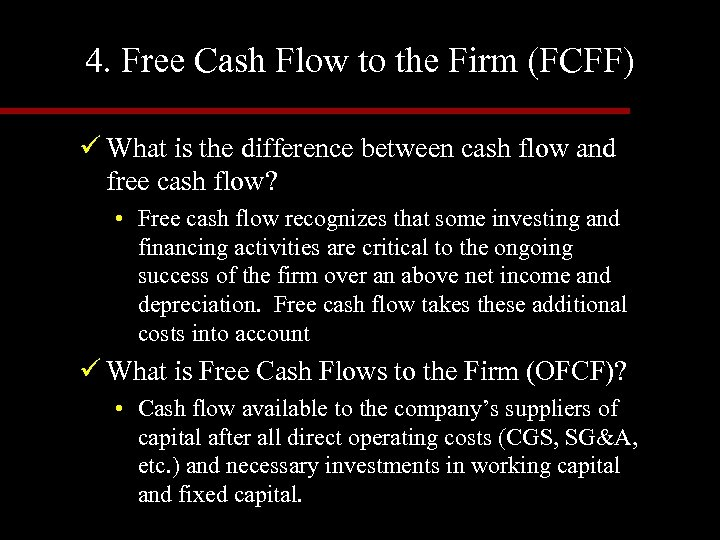4. Free Cash Flow to the Firm (FCFF) ü What is the difference between cash flow and free cash flow? • Free cash flow recognizes that some investing and financing activities are critical to the ongoing success of the firm over an above net income and depreciation. Free cash flow takes these additional costs into account ü What is Free Cash Flows to the Firm (OFCF)? • Cash flow available to the company’s suppliers of capital after all direct operating costs (CGS, SG&A, etc. ) and necessary investments in working capital and fixed capital.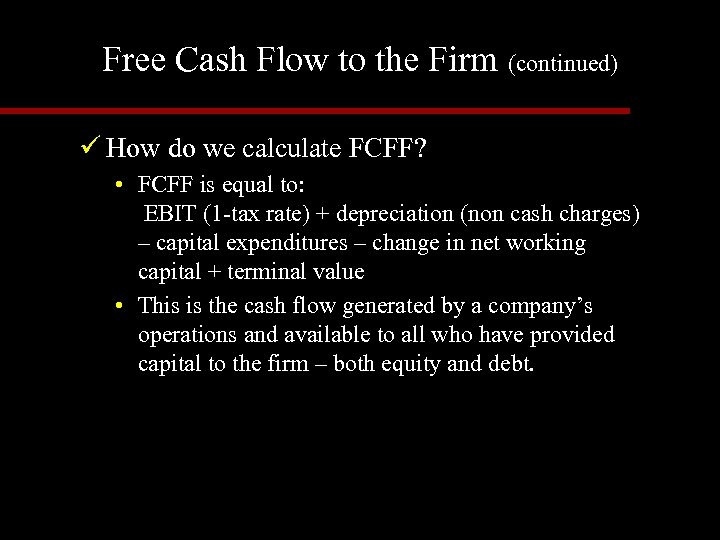Free Cash Flow to the Firm (continued) ü How do we calculate FCFF? • FCFF is equal to: EBIT (1 -tax rate) + depreciation (non cash charges) – capital expenditures – change in net working capital + terminal value • This is the cash flow generated by a company’s operations and available to all who have provided capital to the firm – both equity and debt.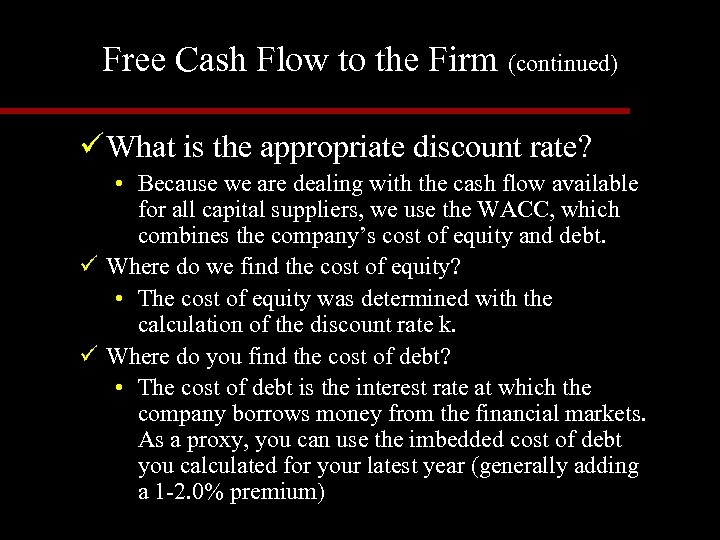Free Cash Flow to the Firm (continued) ü What is the appropriate discount rate? • Because we are dealing with the cash flow available for all capital suppliers, we use the WACC, which combines the company’s cost of equity and debt. ü Where do we find the cost of equity? • The cost of equity was determined with the calculation of the discount rate k. ü Where do you find the cost of debt? • The cost of debt is the interest rate at which the company borrows money from the financial markets. As a proxy, you can use the imbedded cost of debt you calculated for your latest year (generally adding a 1 -2. 0% premium)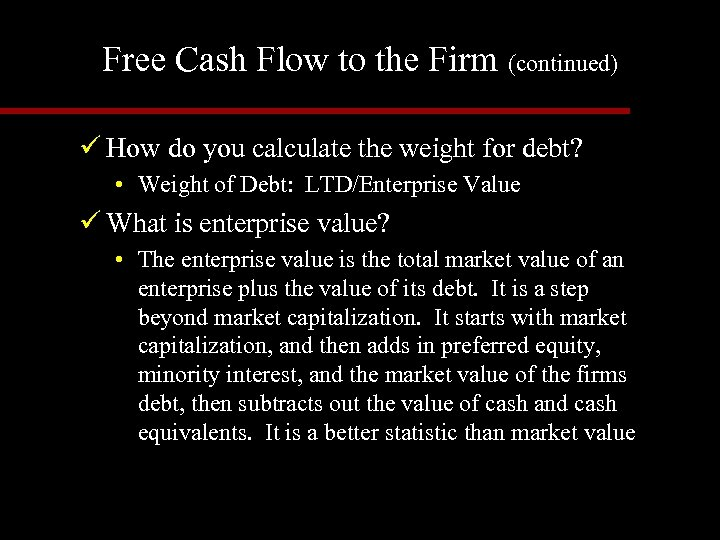Free Cash Flow to the Firm (continued) ü How do you calculate the weight for debt? • Weight of Debt: LTD/Enterprise Value ü What is enterprise value? • The enterprise value is the total market value of an enterprise plus the value of its debt. It is a step beyond market capitalization. It starts with market capitalization, and then adds in preferred equity, minority interest, and the market value of the firms debt, then subtracts out the value of cash and cash equivalents. It is a better statistic than market value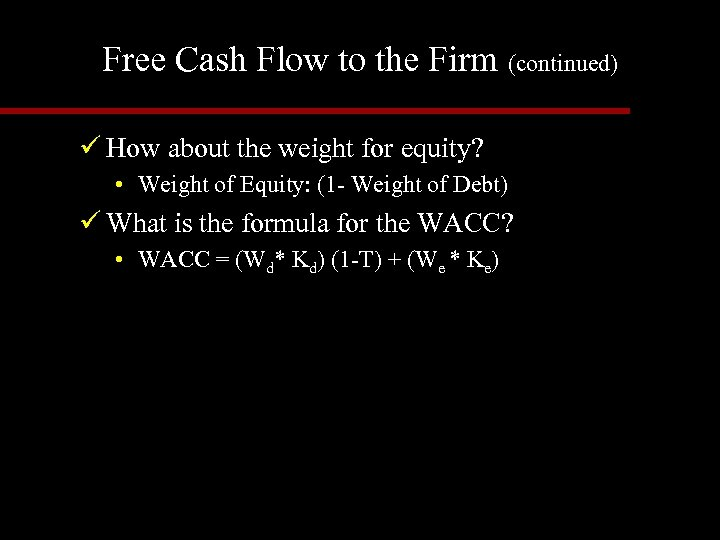Free Cash Flow to the Firm (continued) ü How about the weight for equity? • Weight of Equity: (1 - Weight of Debt) ü What is the formula for the WACC? • WACC = (Wd* Kd) (1 -T) + (We * Ke)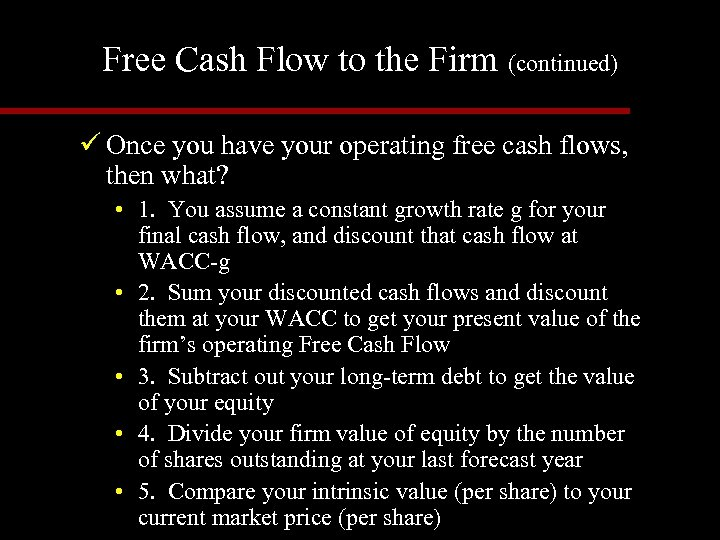Free Cash Flow to the Firm (continued) ü Once you have your operating free cash flows, then what? • 1. You assume a constant growth rate g for your final cash flow, and discount that cash flow at WACC-g • 2. Sum your discounted cash flows and discount them at your WACC to get your present value of the firm’s operating Free Cash Flow • 3. Subtract out your long-term debt to get the value of your equity • 4. Divide your firm value of equity by the number of shares outstanding at your last forecast year • 5. Compare your intrinsic value (per share) to your current market price (per share)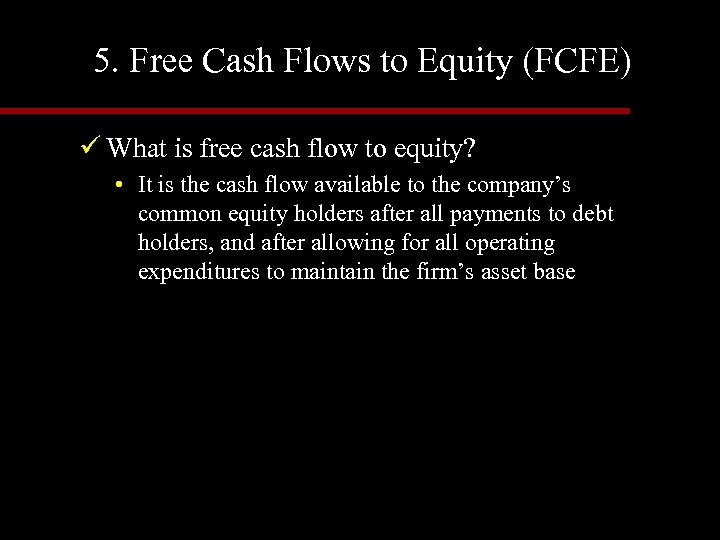5. Free Cash Flows to Equity (FCFE) ü What is free cash flow to equity? • It is the cash flow available to the company’s common equity holders after all payments to debt holders, and after allowing for all operating expenditures to maintain the firm’s asset base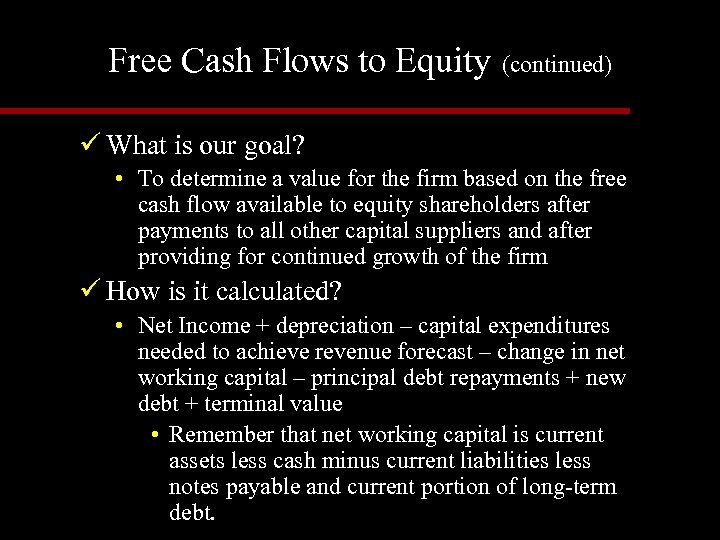Free Cash Flows to Equity (continued) ü What is our goal? • To determine a value for the firm based on the free cash flow available to equity shareholders after payments to all other capital suppliers and after providing for continued growth of the firm ü How is it calculated? • Net Income + depreciation – capital expenditures needed to achieve revenue forecast – change in net working capital – principal debt repayments + new debt + terminal value • Remember that net working capital is current assets less cash minus current liabilities less notes payable and current portion of long-term debt.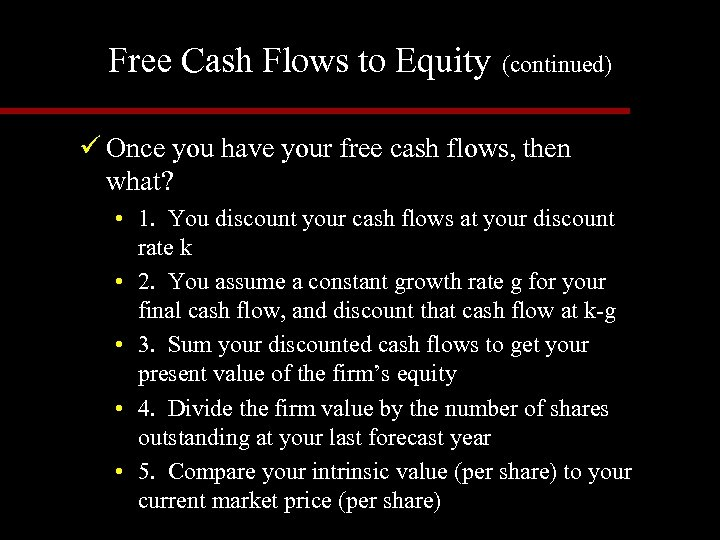Free Cash Flows to Equity (continued) ü Once you have your free cash flows, then what? • 1. You discount your cash flows at your discount rate k • 2. You assume a constant growth rate g for your final cash flow, and discount that cash flow at k-g • 3. Sum your discounted cash flows to get your present value of the firm’s equity • 4. Divide the firm value by the number of shares outstanding at your last forecast year • 5. Compare your intrinsic value (per share) to your current market price (per share)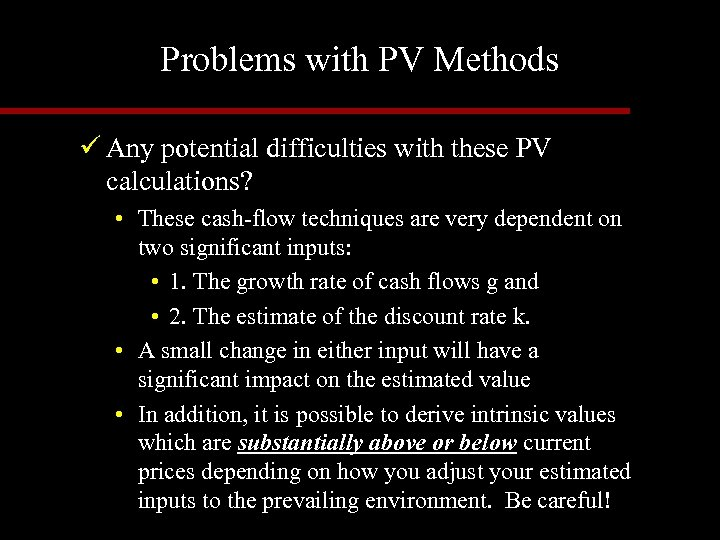Problems with PV Methods ü Any potential difficulties with these PV calculations? • These cash-flow techniques are very dependent on two significant inputs: • 1. The growth rate of cash flows g and • 2. The estimate of the discount rate k. • A small change in either input will have a significant impact on the estimated value • In addition, it is possible to derive intrinsic values which are substantially above or below current prices depending on how you adjust your estimated inputs to the prevailing environment. Be careful!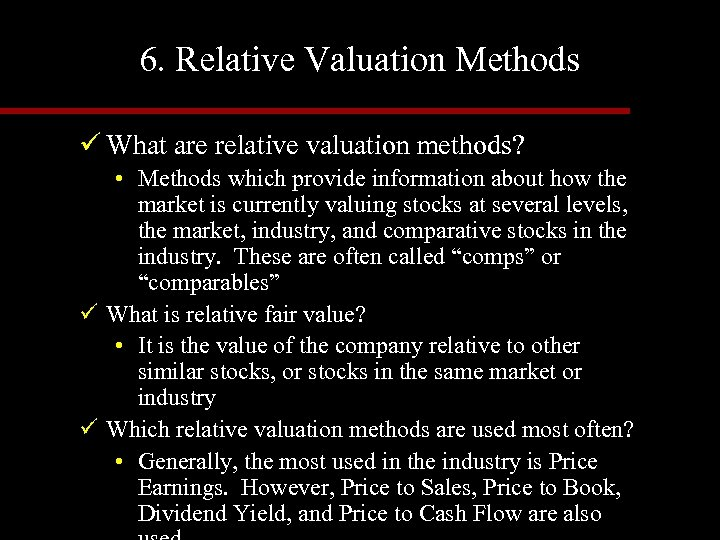6. Relative Valuation Methods ü What are relative valuation methods? • Methods which provide information about how the market is currently valuing stocks at several levels, the market, industry, and comparative stocks in the industry. These are often called “comps” or “comparables” ü What is relative fair value? • It is the value of the company relative to other similar stocks, or stocks in the same market or industry ü Which relative valuation methods are used most often? • Generally, the most used in the industry is Price Earnings. However, Price to Sales, Price to Book, Dividend Yield, and Price to Cash Flow are also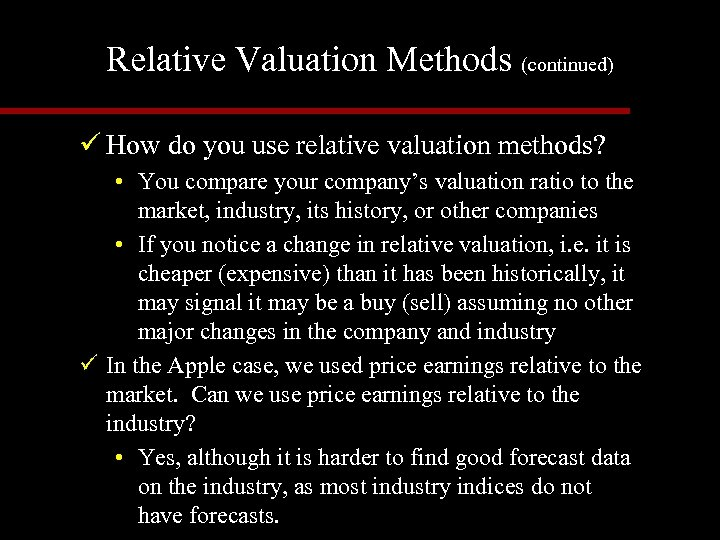Relative Valuation Methods (continued) ü How do you use relative valuation methods? • You compare your company’s valuation ratio to the market, industry, its history, or other companies • If you notice a change in relative valuation, i. e. it is cheaper (expensive) than it has been historically, it may signal it may be a buy (sell) assuming no other major changes in the company and industry ü In the Apple case, we used price earnings relative to the market. Can we use price earnings relative to the industry? • Yes, although it is harder to find good forecast data on the industry, as most industry indices do not have forecasts.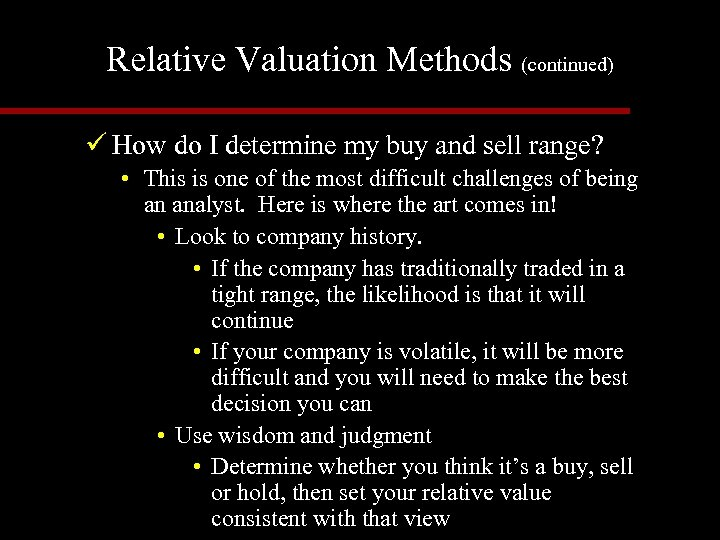Relative Valuation Methods (continued) ü How do I determine my buy and sell range? • This is one of the most difficult challenges of being an analyst. Here is where the art comes in! • Look to company history. • If the company has traditionally traded in a tight range, the likelihood is that it will continue • If your company is volatile, it will be more difficult and you will need to make the best decision you can • Use wisdom and judgment • Determine whether you think it’s a buy, sell or hold, then set your relative value consistent with that view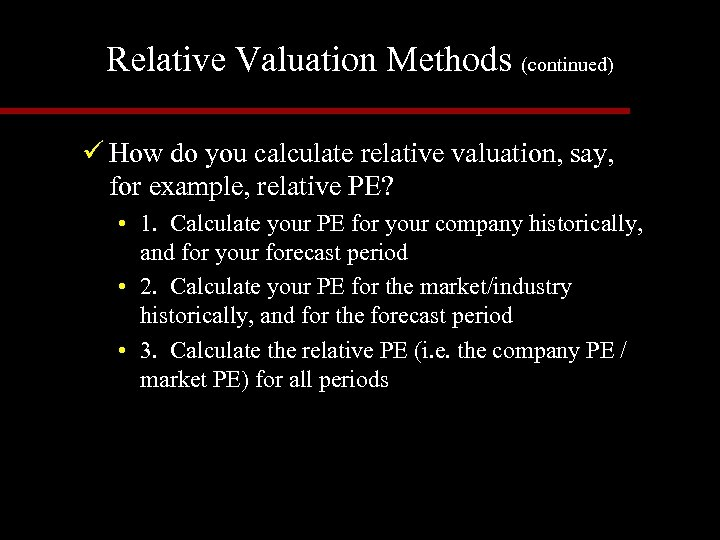Relative Valuation Methods (continued) ü How do you calculate relative valuation, say, for example, relative PE? • 1. Calculate your PE for your company historically, and for your forecast period • 2. Calculate your PE for the market/industry historically, and for the forecast period • 3. Calculate the relative PE (i. e. the company PE / market PE) for all periods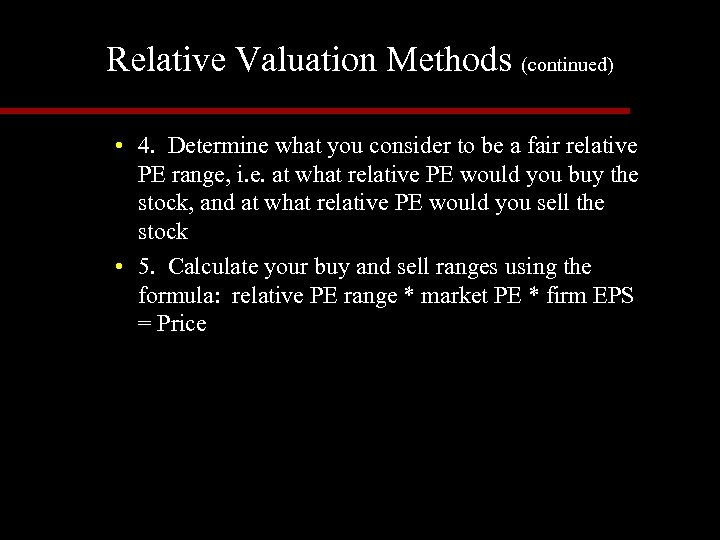Relative Valuation Methods (continued) • 4. Determine what you consider to be a fair relative PE range, i. e. at what relative PE would you buy the stock, and at what relative PE would you sell the stock • 5. Calculate your buy and sell ranges using the formula: relative PE range * market PE * firm EPS = Price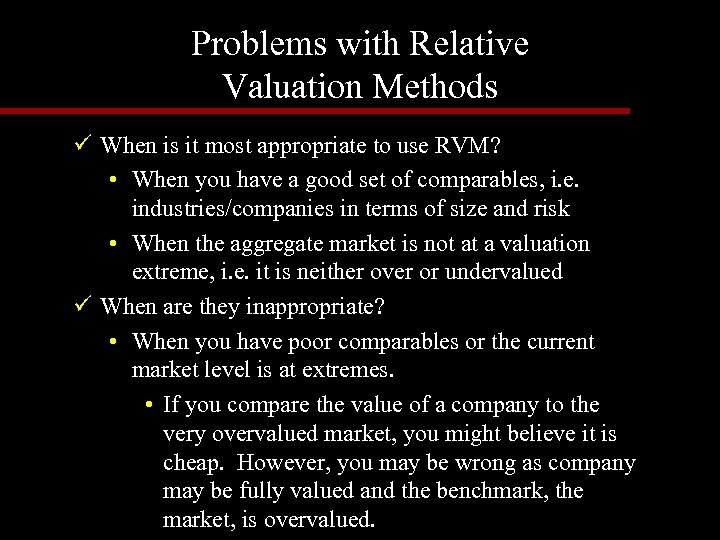Problems with Relative Valuation Methods ü When is it most appropriate to use RVM? • When you have a good set of comparables, i. e. industries/companies in terms of size and risk • When the aggregate market is not at a valuation extreme, i. e. it is neither over or undervalued ü When are they inappropriate? • When you have poor comparables or the current market level is at extremes. • If you compare the value of a company to the very overvalued market, you might believe it is cheap. However, you may be wrong as company may be fully valued and the benchmark, the market, is overvalued.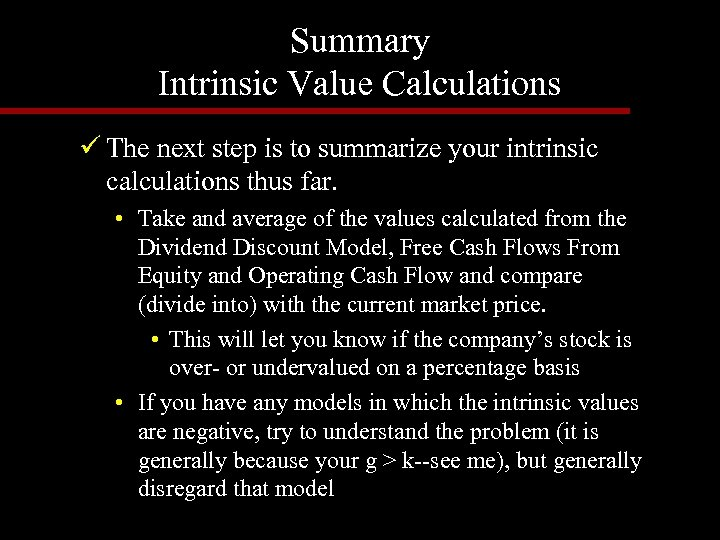Summary Intrinsic Value Calculations ü The next step is to summarize your intrinsic calculations thus far. • Take and average of the values calculated from the Dividend Discount Model, Free Cash Flows From Equity and Operating Cash Flow and compare (divide into) with the current market price. • This will let you know if the company’s stock is over- or undervalued on a percentage basis • If you have any models in which the intrinsic values are negative, try to understand the problem (it is generally because your g > k--see me), but generally disregard that model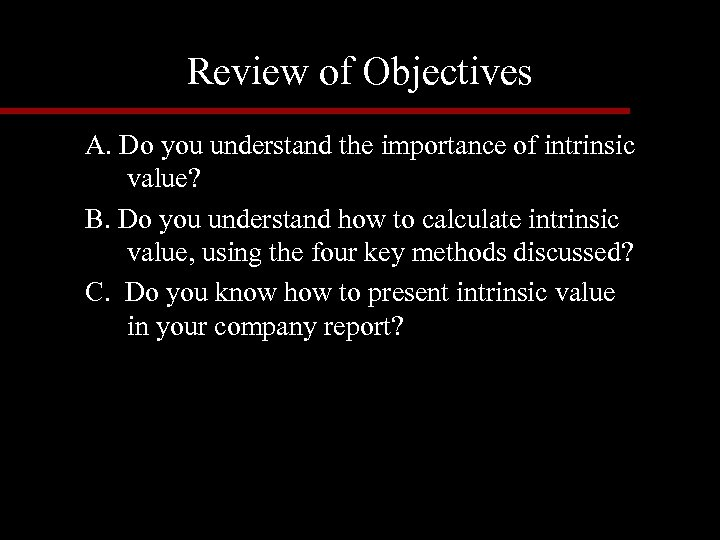Review of Objectives A. Do you understand the importance of intrinsic value? B. Do you understand how to calculate intrinsic value, using the four key methods discussed? C. Do you know how to present intrinsic value in your company report?